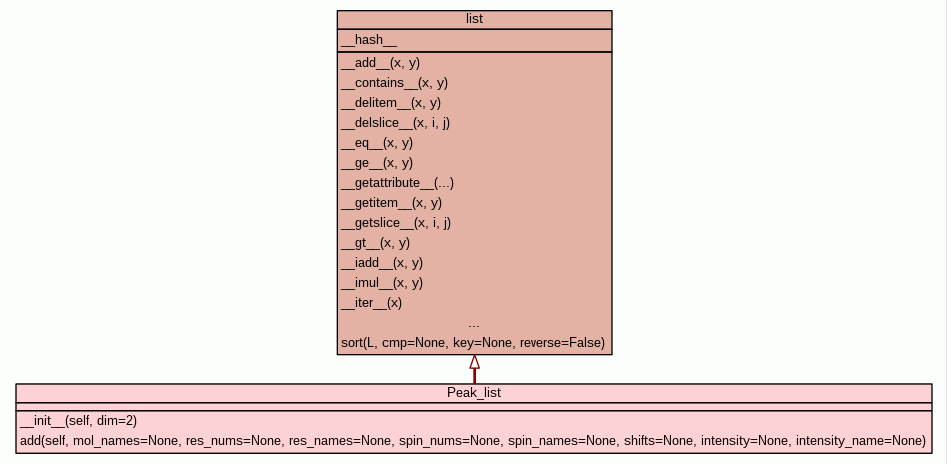[frames] | no frames]

# Class Peak_list

source codeThe object used to represent a peak list.

 Instance Methods
new empty list
 __init__(self, dim=2) Set up the object. source code

 add(self, mol_names=None, res_nums=None, res_names=None, spin_nums=None, spin_names=None, shifts=None, intensity=None, intensity_name=None) Add a peak list element. source code

Inherited from `list`: `__add__`, `__contains__`, `__delitem__`, `__delslice__`, `__eq__`, `__ge__`, `__getattribute__`, `__getitem__`, `__getslice__`, `__gt__`, `__iadd__`, `__imul__`, `__iter__`, `__le__`, `__len__`, `__lt__`, `__mul__`, `__ne__`, `__new__`, `__repr__`, `__reversed__`, `__rmul__`, `__setitem__`, `__setslice__`, `__sizeof__`, `append`, `count`, `extend`, `index`, `insert`, `pop`, `remove`, `reverse`, `sort`

Inherited from `object`: `__delattr__`, `__format__`, `__reduce__`, `__reduce_ex__`, `__setattr__`, `__str__`, `__subclasshook__`

 Class Variables

Inherited from `list`: `__hash__`

 Properties

Inherited from `object`: `__class__`

 Method Details

### __init__(self, dim=2)(Constructor)

source code

Set up the object.

Parameters:
• `dim` (int) - The dimensionality of the peak list.
Returns: new empty list
Overrides: object.__init__

### add(self, mol_names=None, res_nums=None, res_names=None, spin_nums=None, spin_names=None, shifts=None, intensity=None, intensity_name=None)

source code

• `mol_names` (list of str or None) - The list of molecule names for each dimension of the assignment.
• `res_nums` (list of int or None) - The list of residue numbers for each dimension of the assignment.
• `res_names` (list of str or None) - The list of residue names for each dimension of the assignment.
• `spin_nums` (list of int or None) - The list of spin numbers for each dimension of the assignment.
• `spin_names` (list of str or None) - The list of spin names for each dimension of the assignment.
• `shifts` (list of float or None) - The chemical shifts for each dimension of the assignment in ppm.
• `intensity` (list of float or None) - The optional intensity value for the peak. This can have multiple values as some peak lists cover multiple spectra.
• `intensity_name` (list of str or None) - The optional name of the intensity column. This can be used for automated spectrum ID generation.